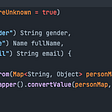# Java streams 24. Reduce

`BiFunction<String, Integer, String> bf1 =         (String s, Integer i) -> {            String r1 = s == null ? "" : s;            String r2 = i == null ? "0" : i.toString();            return r1 + ", " + r2;        };  BiFunction<Integer, String, Integer> bf2 =        (Integer i, String s) -> {            Integer r1 = i == null ? 0 : i;            Integer r2 = s == null ? 0 : s.length();            return r1 + r2;        };`
`System.out.println(bf1.apply("abc", 42));   //prints: abc, 42  System.out.println(bf1.apply(null, 42));    //prints: , 42  System.out.println(bf1.apply("abc", null)); //prints: abc, 0    System.out.println(bf2.apply(42, "abc"));   //prints: 45  System.out.println(bf2.apply(null, "abc")); //prints: 3  System.out.println(bf2.apply(42, null));    //prints: 42`
`  BinaryOperator<String> bo1 =         (String s1, String s2) -> s1 + ", " + s2;  System.out.println(bo1.apply("abc", "42")); //prints: abc, null  System.out.println(bo1.apply(null, "42"));  //prints: null, 42  System.out.println(bo1.apply("abc", null)); //prints: abc, null  BinaryOperator<Integer> bo2 =        (Integer i1, Integer i2) -> {            Integer r1 = i1 == null ? 0 : i1;            Integer r2 = i2 == null ? 0 : i2;            return r1 + r2;        };  System.out.println(bo2.apply(42, 42));     //prints: 84  System.out.println(bo2.apply(null, 42));   //prints: 42  System.out.println(bo2.apply(42, null));   //prints: 42`
`class Box {    int weight;    String color;    public Box(int weight, String color) {        this.weight = weight;        this.color = color;    }    public int getWeight() { return weight; }    public String getColor() { return color; }    @Override    public String toString() {        return "Box{weight=" + weight +                    ", color='" + color + "'}";    }  }`
`Box theHeaviest = Stream.of(new Box(5, "red"),                               new Box(8, "green"),                               new Box(3, "blue"))     .reduce((b1, b2) ->                b1.getWeight() > b2.getWeight() ? b1 : b2)     .orElse(null);  System.out.print(theHeaviest);                   //prints: Box{weight=8, color='green'}`
`BinaryOperator<Box> maxByWeight =       (b1, b2) -> b1.getWeight() > b2.getWeight() ? b1 : b2;  Box theHeaviest = Stream.of(new Box(5, "red"),                               new Box(8, "green"),                               new Box(3, "blue"))           .reduce(maxByWeight)          .orElse(null);  System.out.print(theHeaviest);                       //prints: Box{weight=8, color='green'}`
`BinaryOperator<Box> maxByWeight =        BinaryOperator.maxBy(Comparator                                .comparing(Box::getWeight));`
`int totalWeight = Stream.of(new Box(5, "red"),                               new Box(8, "green"),                               new Box(3, "blue"))        .map(b -> b.getWeight())        .reduce((w1, w2) -> w1 + w2)        .orElse(null);  System.out.print(totalWeight);     //prints: 16`
`String colors = Stream.of(new Box(5, "red"),                             new Box(8, "green"),                             new Box(3, "blue"))        .map(p -> p.getColor())        .reduce((c1, c2) -> c1 + " " + c2)        .orElse(null);  System.out.print(colors);    //prints: red green blue`
`String colors = Stream.of(new Box(5, "red"),                             new Box(8, "green"),                             new Box(3, "blue"))        .map(p -> p.getColor())        .reduce((c1, c2) -> c1 + ", " + c2)        .orElse(null);  System.out.print(colors);    //prints: red, green, blue`
`List<Box> list = List.of(new Box(5, "red"),                            new Box(8, "green"),                            new Box(3, "blue"));  StringBuffer sb = new StringBuffer();  int count = 1;  for(Box b: list){    sb.append(b.getColor());    if(count < list.size()){        sb.append(", ");    }    count++;  }  System.out.print(sb.toString());  //prints: red, green, blue`
`int totalWeight5 = Stream.of(new Box(5, "red"),                                new Box(8, "green"),                                new Box(3, "blue"))        .map(b -> b.getWeight())        .reduce(10, (w1, w2) -> w1 + w2);  System.out.print(totalWeight5);      //prints: 26`
`String colors = Stream.of(new Box(5, "red"),                             new Box(8, "green"),                             new Box(3, "blue"))        .map(p -> p.getColor())        .reduce("Colors: ", (c1, c2) -> c1 + " " + c2);  System.out.print(colors);                  //prints: Colors: red green blue`
`String colors = Stream.of(new Box(5, "red"),                             new Box(8, "green"),                             new Box(3, "blue"))        .parallel()        .map(p -> p.getColor())        .reduce("Colors:", (c1, c2) -> c1 + " " + c2,                            (r1, r2) -> r1 + " " + r2);  System.out.print(colors);         //prints: Colors: red Colors: green Colors: blue`
`String colors = Stream.of(new Box(5, "red"),                            new Box(8, "green"),                            new Box(3, "blue"))        .parallel()        .map(p -> p.getColor())        .reduce("Colors:", (c1, c2) -> c1 + " " + c2,              (r1, r2) -> r1 + " " + r2.replace("Colors: ", ""));  System.out.print(colors);     //prints: Colors: red green blue`
`int sum = Stream.of(1, 2, 3)        .parallel()        .reduce(0, (i1, i2) -> i1 + i2,                    (r1, r2) -> r1 + r2);   System.out.print(sum);     //prints: 6`
`int sum = Stream.of(1, 2, 3)        .parallel()        .reduce(0, Integer::sum, Integer::sum);  System.out.print(sum);    //prints: 6`
`BiFunction<List<String>, String, List<String>> accumulator =        (l, s) -> {            l.add(s);            return l;        };  BinaryOperator<List<String>> combiner =        (l1, l2) -> {            //Does not do anything except printing:            System.out.println("In combiner!");            return l1;        };  List<String> lst = Stream.of("a", "b", "c", "d", "e")        .reduce(new ArrayList<>(), accumulator, combiner);  System.out.print(lst);            //prints: [a, b, c, d, e]`

--

--

--

## More from Nick Samoylov

Born in Moscow, lived in Crimea, now lives in the US. Used to be physicist and rock climber, now programmer and writer.

Love podcasts or audiobooks? Learn on the go with our new app.

## How to deal with Conda Environments## Concurrency in Go is Not Magic## Just Enough Functional Programming## How To Efficiently Use Common Meta Tags for Your Website?## Distributed Transaction## Nick Samoylov

Born in Moscow, lived in Crimea, now lives in the US. Used to be physicist and rock climber, now programmer and writer.

## Java record: A Brief## Functional Java CodeStyle [Part: 3]## Visitor Design Pattern in Java